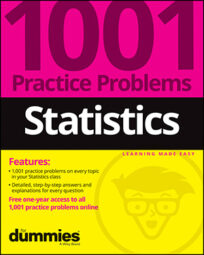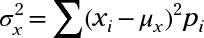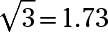##### Statistics: 1001 Practice Problems For Dummies (+ Free Online Practice)Use the following formula for the variance of a discrete random variable X as needed to answer the following problems (round each answer to two decimal places):## Sample questions

1. If the variance of a discrete random variable X is 3, what is the standard deviation of X?

The standard deviation is the square root of the variance, so if the variance of X is 3, the standard deviation of X is(rounded to two decimal places).

2. If the standard deviation of X is 0.65, what is the variance of X?